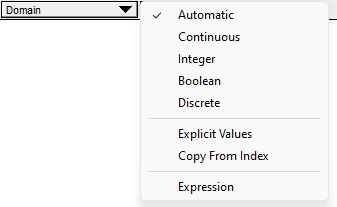# Domain attribute

Release: 4.6  •  5.0  •  5.1  •  5.2  •  5.3  •  5.4  •  6.0  •  6.1  •  6.2  •  6.3  •  6.4

## What is the Domain Attribute?

The Domain attribute specifies the type or range of valid values for a variable, and how those values should be interpreted -- e.g., as discrete or continuous.## Continuous vs. Discrete

The possible values for a numeric value may be continuous or discrete. For example, a variable such as Number_of_failures is integer-valued, and is therefore "discrete numeric", while a variable such as Airspeed is real-valued and thus continuous.

When dealing with uncertain numeric quantities, knowing whether a variable is continuous or discrete is necessary for the proper statistical treatment. When expressing uncertainty, we use probability of outcomes for discrete-valued variables, and probability-densities for continuous variables.

### Where is this used

When Analytica computes or graphs statistical results, the appropriate computation changes depending on whether the quantity is discrete or continuous. For example, Pdf returns a 1-D array of probabilities, indexed by a .Possible_Values index, for a discrete variable, but for a continuous variable it returns a two-D array indexed by .Step and DensityIndex. Results of Frequency, GetFract, Pdf, and Cdf are all influenced by this distinction.

The domain also determines the space of options for Slider and self-Choice controls.

### Automatic

When the domain is set to Automatic, Analytica attempts to infer whether a variable is discrete or continuous based on the values that occur. This inference is heuristic -- it is a guess on Analytica's part. Most the time, Analytica will guess correctly. For example, if the data contains a small number of unique integer values, as might result from a Poisson or Geometric distribution, Analytica will usually infer that the domain is discrete, while if many real-valued quantities occur, as with a Normal distribution, it will correctly infer that the quantity is continuous.

However, because the inference is heuristic, it may get it wrong in some cases. When this happens, or proactively to prevent this possibility, you can explicitly set the domain to indicate whether the quantity is discrete or continuous.

### Continuous

Select a Continuous domain if your quantity is real-valued.

### Discrete Numeric

Select this if only certain discrete values are possible, as with an integer-valued quantity. If you can identify the exact set of possible values, consider setting an explicit domain, as either a list of numbers, or as an index-valued domain.

### Categorical

If your variable may have non-numeric values, or you do not wish the value to be interpreted numerically, set the domain to Categorical.

## Explicit Domains

If you can identify a finite set of possible values, you may list these explicitly using a List, List-of-labels, or Index domain.

When your variable contains an explicit domain, it can serve as an index or a value. When an expression occurs in an index context, the domain value is used. Furthermore, the variable can be used to index a DetermTable or Probability Table.

### Index-Domain

An index domain uses the index values of another indicated index variable. If the possible values already exists in another index, or if many variables share the same domain, the values only have to be listed once. Furthermore, with this feature, values can be computed (the evaluated index values are used).

The domain attribute uses the evaluated value of the original index. This, the following two examples do not have equivalent domains:

Example A:

Index I := [1 + 2, cos(45), X]
Domain of D := Index I

Example B:

Domain of D := [1 + 2, cos(45), X]

Assume X = 2.1. In example A, the domain of D is [3, 0.707, 2.1], while in Example B it is a 3-element list with expressions 1 + 2, cos(45), and the varTerm X.

Likewise, the domain of D can be set to a variable that is defined as an expression that returns a list when evaluated.